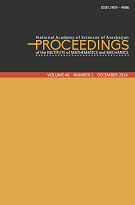﻿ Volume 48, Issue 1 — Proc. Inst. Math. Mech.P R O C E E D I N G S
of the
Institute of Mathematics and Mechanics

 ISSN 2409-4986 (Print) ISSN 2409-4994 (Online)

## Volume 48, Issue 1

An inverse problem for the quadratic pencil of differential operators with almost periodic coefficients
by Ashraf D. Orujov

Symmetry solutions and conserved vectors for a generalized short pulse equation
by Chaudry Masood Khalique and Anila Mehmood

Phragmen-Lindelof theorem for a class of non-uniformly elliptic equation
by Farman Mamedov and Narmin Amanova

Infimal Convolution and Duality in Convex Mathematical Programming
by Elimhan N. Mahmudov and Misir J. Mardanov

On well-defined solvability of the Dirichlet problem for a second order elliptic partial operator-differential equation in Hilbert space
by Hamidulla I. Aslanov and Roya F. Hatamova

$A$-Berezin number of operators
by Mehmet Gurdal and Hamdullah Basaran

On substitution and extension operators in Banach-Sobolev function spaces
by Eminaga M. Mamedov

To the inverse spectral problem for a perturbed oscillator on the semiaxis

On some asymptotically half-linear eigenvalue problem for ordinary differential equations of fourth order

Boundedness of the Discrete Ahlfors-Beurling Transform on discrete Morrey spaces
by Rashid A. Aliev, Aynur N. Ahmadova and Aynur F. Huseynli

On a new parameterized Beta Function
by Mustapha Ra\"{\i}ssouli and Mohamed Chergui

A formula for the approximation of functions by single hidden layer neural networks with weights from two straight lines
by Vugar E. Ismailov

Optimization of source parameters in non-local boundary conditions of a large system of ODE
by Yegana R. Ashrafova

Exploring new features for the perturbed Chen-Lee-Liu model via $(m+\frac{1}{G'})$-expansion method
by Hasan Bulut and Hajar F. Ismael

 © 2023 Institute of Mathematics and Mechanics